### PMT Function Examples – Excel, VBA, & Google Sheets

This tutorial demonstrates how to use the Excel PMT Function in Excel to calculate the periodic payment amount of an investment.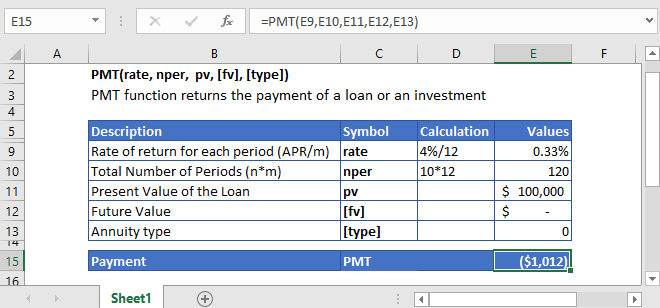## PMT Function Overview

The PMT Function Calculates the payment amount.

To use the PMT Excel Worksheet Function, select a cell and type: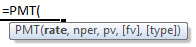(Notice how the formula inputs appear)

### PMT Function Syntax and Inputs:

`=PMT(rate,nper,pv,[fv],[type])`

rate – The interest rate for each period.

nper – The total number of payment periods.

pv – The present value of the investment that the future payments are worth now.

fv – OPTIONAL: The Future Value of the investment or loan at the end of the number of payment periods.

type – OPTIONAL: The type argument shows when the payments are made, either at the end of the period by 0 or at the start of the period by 1. Its default value is 0.

AutoMacro - VBA Code Generator

## What Is PMT?

Periodic Payments or simply the payments are such payments that are made consistently for a number of periods to pay off the loan or investment. The periodic payments can be calculated from either using the present value formula or future value formula.

If the Present Value is given then the PMT is calculated using the following equation:

`PMT = PV/[(1-1/(1 +R)^N )/R]`

If the Future Value of the investment is given then PMT is calculated using this equation:

`PMT = FV/[(1/(1 +R)^N -1)/R]`

Where:

PV = Present Value

FV = Future Value

R = Rate of Return

N = Total Number of periods

## What is the Excel PMT Function?

Excel PMT function calculates the total periodic payments made against an investment or loan at a constant interest rate for a specific number of periods. The periodic payments, PMT, can be made at the beginning of the period or the end of the period. By default, the excel function considered that the payments are made at the end of the period.

### Calculate Monthly Payments of a Loan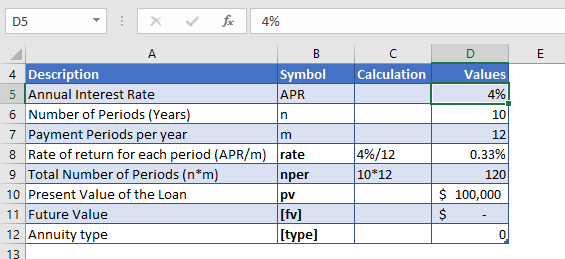Let’s assume that a student loan of \$100,000 is to be paid off completely in 10 years with a 4% annual interest rate. The monthly payment of the loan has to be calculated.

As the payments are made monthly, the annual interest rate is converted into monthly interest by

Monthly Interest Rate – 4% (annual interest rate) / 12 (months per year) = 0.33%

and the number of payments per period is converted into the monthly number of payments by

NPER – 10 (years) * 12 (months per year) = 120

The formula used for the calculation of the payments is:

`=PMT(D10,D11,D12,D13,D14)`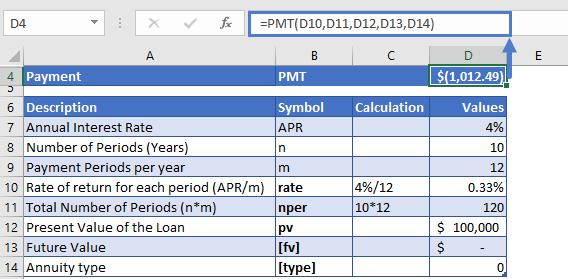The monthly payment of the loan is

PMT = -\$1,012.

The result came in negative value because it shows that the cash is being paid out from the individual account.

### Calculate Monthly Payments for a Savings account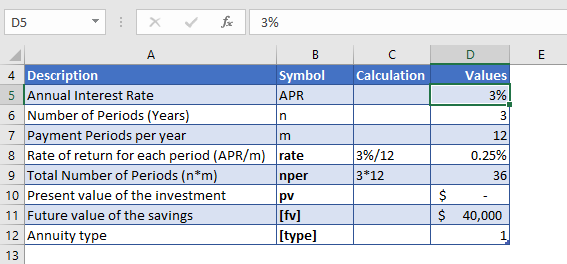Suppose you want to buy a car for three years now. So you open a savings account intending to reach \$40,000 by the end of the three years. You want to find out the monthly payments that you need to make. And consider the bank pays you an annual interest rate of 3% on your payments.

The payments are made monthly, so the annual interest rate is converted into monthly interest by

Monthly Interest Rate – 3% (annual interest rate) / 12 (months per year) = 0.25%

and the number of payments per period is converted into the monthly number of payments by

NPER – 3 (years) * 12 (months per year) = 36

Here, the present value of the investment is not given, so we entered the following value in the pv argument

PV = 0

The formula used for the calculation is:

`=PMT(D10,D11,D12,D13,D14)`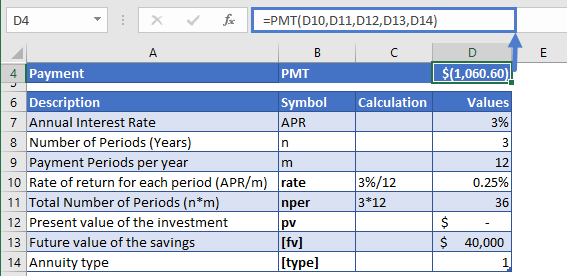The monthly installment is

PMT = -\$1061

The result came in negative value as the payments are being made out to the bank (from own’s account) every month.

## Additional Notes

Make sure the units of nper and rate are consistent, i.e. in case of monthly interest rate the number of periods of investment should also be in months.

In the Excel Financial Functions the cash outflows, such as deposits, are represented by negative numbers and the cash inflows, such as dividends, are represented by positive numbers.

Return to the List of all Functions in Excel

## PMT in Google Sheets

All of the above examples work exactly the same in Google Sheets as in Excel.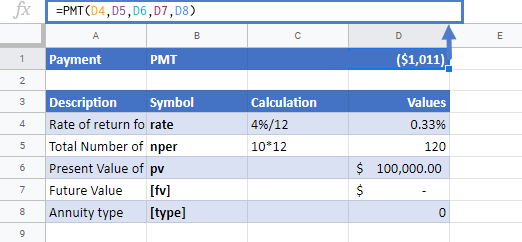## PMT Examples in VBA

You can also use the PMT function in VBA. Type:
`application.worksheetfunction.pmt(rate,nper,pv,fv,type)`
For the function arguments (rate, etc.), you can either enter them directly into the function or define variables to use instead.

## Excel Practice Worksheet

Practice Excel functions and formulas with our 100% free practice worksheets!

• Automatically Graded Exercises
• Learn Excel, Inside Excel!